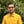Trusted answers to developer questions
Trusted Answers to Developer Questions

Related Tags

python
math
communitycreator

# What is math.asin() in Python?Harris Amjad

Grokking Modern System Design Interview for Engineers & Managers

Ace your System Design Interview and take your career to the next level. Learn to handle the design of applications like Netflix, Quora, Facebook, Uber, and many more in a 45-min interview. Learn the RESHADED framework for architecting web-scale applications by determining requirements, constraints, and assumptions before diving into a step-by-step design process.

The asin() functionalso called the arc sine function returns the inverse sine of a number. To be more specific, it returns the inverse sine of a number in radians.

Figure 1 shows the mathematical representation of the asin() function, and Figure 2 shows the visual representation of the asin() function.

Figure 1: Mathematical representation of inverse sine function
Figure 2: Visual representation of inverse sine function

Note: The math module is required for this function.

To convert radians to degrees, use:

degrees = radians * ( 180.0 / pi )


## Syntax

asin(num)


## Parameter

This function requires a number as a parameter. The parameter must be a double value between -1 and 1.

• -1 <= parameter <= 1

## Return value

asin() returns the inverse sine of a numberradians that is sent as a parameter. The return value lies in interval [-pi/2, pi/2] radians.

## Example

import math#positive number in radiansprint "The value of asin(0.5) : ",  math.asin(0.5), "Radians"#negative number in radiansprint "The value of asin(-0.5) : ",  math.asin(-0.5), "Radians"#applying asin() and then converting the result in radians to degrees#radians = 0.5#PI = 3.14159265result = math.asin(0.5) * (180.0 / math.pi)print "The value of asin(0.5) : ", result ,"Degrees"

RELATED TAGS

python
math
communitycreator

CONTRIBUTORHarris Amjad

Grokking Modern System Design Interview for Engineers & Managers

Ace your System Design Interview and take your career to the next level. Learn to handle the design of applications like Netflix, Quora, Facebook, Uber, and many more in a 45-min interview. Learn the RESHADED framework for architecting web-scale applications by determining requirements, constraints, and assumptions before diving into a step-by-step design process.

Keep Exploring

Learn in-demand tech skills in half the time